In this article, we will share MP Board Class 10th Maths Book Solutions Chapter 2 Polynomials Ex 2.3 Pdf, These solutions are solved subject experts from the latest edition books.

## MP Board Class 10th Maths Solutions Chapter 2 Polynomials Ex 2.3

Question 1.
Divide the polynomial p(x) by the polynomial g(x) and find the quotient and remainder in each of the following:
(i) p(x) = x3 – 3x2 + 5x – 3, g(x) = x2 – 2
(ii) p(x) = x4 – 3x2 + 4x + 5, g(x) = x2 + 1 – x
(iii) p(x) = x4 – 5x + 6, g(x) = 2 – x2
Solution:
(i) Here, dividend p(x) = x3 – 3x2 + 5x – 3, and divisor g(x) = x2 – 2
∴ We have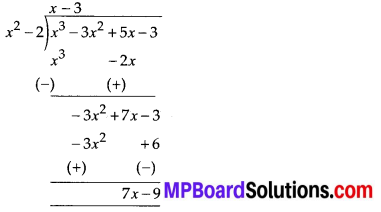Thus, the quotient = (x – 3) and remainder = (7x – 9)

(ii) Here, dividend p(x) = x4 – 3x2 + 4x + 5 and divisor g(x) = x2 + 1 – x = x2 – x + 1
∴ We have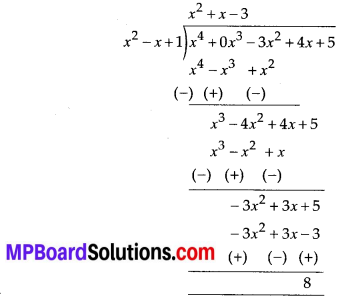Thus, the quotient = (x2 + x – 3) and remainder = 8

(iii) Here, dividend, p(x) = x4 – 5x + 6 and divisor, g(x) = 2 – x2 = – x2 + 2
∴ We have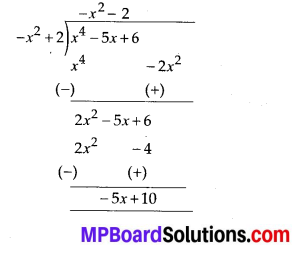Thus, the quotient = -x2 – 2 and remainder = -5x +10Question 2.
Check whether the first polynomial is a factor of the second polynomial by dividing the second polynomial by the first polynomial:
(i) t2 – 3; 2t4 + 3t3 -2t2 – 9t – 12
(ii) x2 + 3x + 1; 3x4 + 5x3 – 7x2 + 2x +2
(iii) x3 – 3x + 1; x5 – 4x3 + x2 + 3x + 1
Solution:
(i) Dividing 2t4 + 3t3 – 2t2 – 9t – 12 by t2 – 3, we have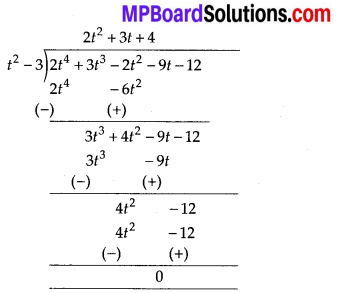∵ Remainder = 0
∴ (t2 – 3) is a factor of 2t4 + 3t3 – 2t2 – 9t – 12.

(ii) Dividing 3x4 + 5x3 – 7x2 + 2x + 2 by x2 + 3x + 1, we have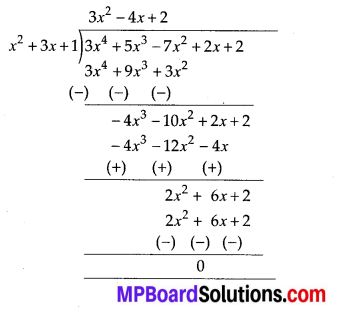∵ Remainder = 0
∴ x2 + 3x + 1 is a factor of 3x4 + 5x3 – 7x2 + 2x + 2.

(iii) Dividing x5 – 4x3 + x2 + 3x + 1 by x3 – 3x + 1, we get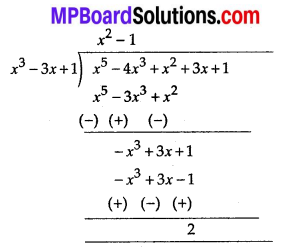∵ Remainder = 2, i.e., remainder = 0
∴ x3 – 3x + 1 is not a factor of x5 – 4x3 + x2 + 3x +1.Question 3.
Obtain all other zeroes of 3x4 + 6x3 – 2x2 – 10x – 5, if two of its zeroes are $$\sqrt{\frac{5}{3}}$$ and $$-\sqrt{\frac{5}{3}}$$
Solution:
We have p(x) = 3x4 + 6x3 – 2x2 – 10x – 5.
Given $$\sqrt{\frac{5}{3}}$$ and $$-\sqrt{\frac{5}{3}}$$ are zeroes of p(x).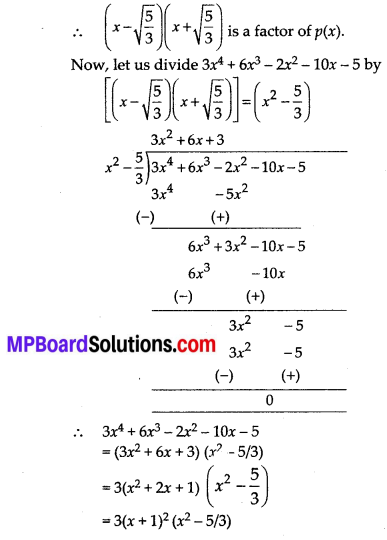Thus, the other zeroes of the given polynomial are -1 and -1.Question 4.
On dividing x3 – 3x2 + x + 2 by a polynomial g(x), the quotient and remainder were x – 2 and -2x + 4, respectively. Find g(x).
Solution:
Here, dividend, p(x) = x3 – 3x2 + x + 2, divisor = g(x), quotient = (x – 2) and remainder = (-2x + 4)
Since, (Quotient × Divisor) + Remainder = Dividend
∴ [(x – 2) × g(x)] + [(-2x + 4)] = x3 – 3x2 + x + 2
⇒ (x – 2) × g(x)
= x3 – 3x2 + x + 2 – (-2x + 4)
= x3 – 3x2 + x + 2 + 2x – 4
= x3 – 3x2 + 3x – 2
= x3 – 3x2 + 3x – 2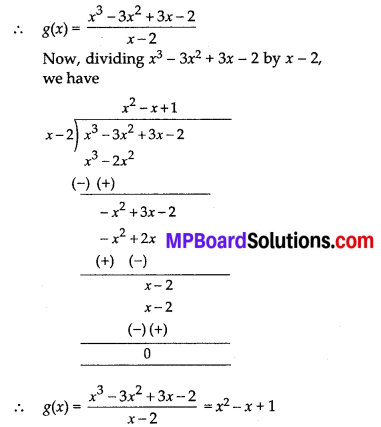Thus, the required divisor g(x) = x2 – x + 1Question 5.
Give examples of polynomials p(x), g(x), q(x) and r(x), which satisfy the division algorithm and
(i) deg p(x) = deg q(x)
(ii) deg q(x) = deg r(x)
(iii) deg r(x) = 0
Solution:
(i) p(x) = 3x2 – 6x + 27,
g(x) = 3 and q(x) = x2 – 2x + 9.
Now, deg p(x) = deg q(x)
r(x) = 0
⇒ p(x) = q(x) × g(x) + r(x)

(ii) p(x) = 2x3 – 2x2 + 2x + 3,
g(x) = 2x2 – 1, and
r(x) = 3x + 2, deg q(x) = deg r(x)
⇒ p(x) = q(x) × g(x) + r(x)

(iii) p(x) = 2x3 – 4x2 + x + 4,
g(x) = 2x2 + 1,
q(x) = x – 2 and r(x) = 6,
deg r(x) = 0
⇒ p(x) = q(x) × g(x) + r(x)